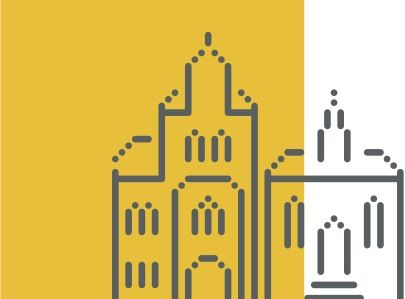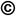TOP
##### Suche auf der Schloss Dagstuhl Webseite
Sie suchen nach Informationen auf den Webseiten der einzelnen Seminare? - Dann:
Nicht fündig geworden? - Einige unserer Dienste laufen auf separaten Webseiten mit jeweils eigener Suche. Bitte beachten Sie folgende Liste:##### Seminare
###### Externe Seiten:
• DOOR (zum Registrieren eines Dagstuhl Aufenthaltes)
• DOSA (zum Beantragen künftiger Dagstuhl Seminare oder Dagstuhl Perspektiven Workshops)
##### dblp
###### Externe Seiten:
• die Informatik-Bibliographiedatenbank dblp

### The Graph Isomorphism Problem

##### ( 13. Dec – 18. Dec, 2015 )

(zum Vergrößern in der Bildmitte klicken)

Bitte benutzen Sie folgende Kurz-Url zum Verlinken dieser Seite: https://www.dagstuhl.de/15511

##### Organisatoren
• (University of Chicago, US)
• (University of Cambridge, GB)
• (RWTH Aachen, DE)
• (Universität Ulm, DE)

##### Motivation

The Graph Isomorphism Problem remains one of the few natural computational problems with unknown complexity status. In very rough terms the problem is to decide whether two given graphs are structurally different or one is just a perturbed variant of the other.

The problem naturally arises when one is faced with the task of classifying relational structures. Having been open and quite prominent for some time now, the Graph Isomorphism Problem is repeatedly attacked with the abundance of algorithmic techniques that have been developed over the decades. While this has not led to a resolution of the problem it has led to applications of techniques developed for the Graph Isomorphism Problem in other areas (such as machine learning and constraint satisfaction problem solving) as well as sparked fascinating concepts in complexity theory, led to a thriving compilation of techniques in algorithmic group theory, the development of software packages (such as canonical labeling tools) and perpetuating effects in algorithmic graph theory in general.

While many other computational problems have a canonical community associated with them, resulting in dedicated conferences, the situation for the isomorphism problem is different. This is due to the fact that the background of people working on the isomorphism problem is quite diverse which leads to infrequent encounters at regular conferences or other events.

This diverse landscape manifests itself for example in the following communities:

• practitioners that implement software around the Graph Isomorphism Problem yielding widely used packages and libraries
• logicians approaching the Graph Isomorphism Problem with techniques such as descriptive complexity theory, and using the problem to prove lower bounds in several proof systems
• algorithmic group theorists that drive the approach to the problem via group theory, yielding to date the best known algorithm with respect to worst-case complexity
• graph theoristsanalyzing the underlying objects from a structural viewpoint solving ever-increasing subcases of the general problem
• complexity theorists highlighting the special position the Graph Isomorphism Problem takes in the complexity landscape
• scientists from the area of combinatorial optimization that apply optimization techniques to isomorphism solving (such as semi-definite programming hierarchies) and conversely use isomorphism tools to speed up optimization procedures
• researchers that apply isomorphism related techniques in and from other areas such as machine learning

In this seminar we bring together researchers working on these core facets of the Isomorphism Problem. This bridges gaps between theory and practice, by exposing practical bottlenecks to theoreticians on the one hand and encouraging the use of new theoretical techniques in practice on the other hand. It also fosters collaboration among the theorists allowing the combination of orthogonal approaches. This will help to push the boundary of our understanding, bringing us hopefully a step closer to understanding the Graph Isomorphism Problem.

The program will include the following five overview talks on topics of great current interest.

1. Graph indistinguishability through hierarchies of relaxations [Albert Atserias]
2. Practical aspects of the graph isomorphism problem [Brendan McKay]
3. Graph isomorphism: the bottlenecks [Laszlo Babai]
4. Complexity classes and graph isomorphism [Jacobo Toran]
5. Parameterizations and the graph isomorphism problem [Pascal Schweitzer]

### Press Reviews

##### Summary

The Graph Isomorphism Problem remains one of the two unresolved computational problems from Garey and Johnson's list dating back to 1979 of problems with unknown complexity status. In very rough terms the problems asks to decide whether two given graphs are structurally different or one is just a perturbed variant of the other. The problem naturally arises when one is faced with the task of classifying relational structures (e.g., chemical molecules, websites and links, road networks).

While the Graph Isomorphism Problem was intensively studied from the point of view of computational complexity in the 1980s and early 1990s, in later years progress became slow and interest in the problem stalled. However, recent years have seen the emergence of a variety of results related to graph isomorphism in a number of research areas including algorithmic group theory, finite model theory, combinatorial optimization and parameterized algorithms, not to mention graph theory itself. Indeed, having been open and quite prominent for such a long time, the Graph Isomorphism Problem is repeatedly attacked with the abundance of algorithmic techniques that have been developed over the decades. While this has not led to resolution of the problem, it has led to applications of methods originally developed for the Graph Isomorphism Problem in other areas (such as machine learning and constraint satisfaction problem solving). It has also sparked fascinating concepts in complexity theory, led to a thriving compilation of techniques in algorithmic group theory, the development of software packages (such as canonical labeling tools) and perpetuating effects in algorithmic graph theory in general.

While a lot of other computational problems have a specific community associated with them, resulting in dedicated conferences, the situation for the isomorphism problem is different. This is due to the fact that the background of people working on the isomorphism problem is quite diverse which leads to infrequent encounters at regular conferences or other events. Moreover, there is a big gap between theory and practice, a phenomenon verbalized by Brendan McKay as two distinct galaxies with very few stars in between them. Indeed, the algorithms that are asymptotically fastest in theory are very different to the ones that prove to be the fastest in practical implementations. The original motivation of the seminar was to bring together researchers working on the many topics closely related to the Isomorphism Problem to foster their collaboration.

However, the face of the seminar was to change, as one of the organizers (László Babai) published a proof on the arXiv (http://arxiv.org/abs/1512.03547) on the night before the seminar that shows that graph isomorphism can be solved in quasi-polynomial time (see the abstract to the talk below). This is the first improvement over the moderately exponential algorithm for general graphs by Luks from 1983. Babai gave three intense blackboard presentations each with a duration of two hours on the new quasi-polynomial time algorithm. Apart from the presentations, there were a number of excellent talks including expository surveys on recent advances in a variety of aspects of the Graph Isomorphism Problem as detailed below.

Overall a memorable event, we hope that the seminar has encouraged future collaboration across the different areas which eventually brings us closer to the theoretical and practical resolution of the problem.Laszlo Babai, Anuj Dawar, Pascal Schweitzer, and Jacobo Torán

##### Teilnehmer
• Albert Atserias (UPC - Barcelona, ES) [dblp]
• Laszlo Babai (University of Chicago, US) [dblp]
• Christoph Berkholz (HU Berlin, DE) [dblp]
• Jannis Bulian (University of Cambridge, GB) [dblp]
• Sourav Chakraborty (Chennai Mathematical Institute, IN) [dblp]
• Maurice Chandoo (Leibniz Universität Hannover, DE) [dblp]
• Bireswar Das (Indian Institute of Technology Gandhinager, IN) [dblp]
• Anuj Dawar (University of Cambridge, GB) [dblp]
• Michael Elberfeld (RWTH Aachen, DE) [dblp]
• Martin Fürer (Pennsylvania State University - University Park, US) [dblp]
• Martin Grohe (RWTH Aachen, DE) [dblp]
• Rohit Gurjar (FH Aalen, DE) [dblp]
• Neil Immerman (University of Massachusetts - Amherst, US) [dblp]
• Tommi Junttila (Aalto University, FI) [dblp]
• Sandra Kiefer (RWTH Aachen, DE) [dblp]
• Johannes Köbler (HU Berlin, DE) [dblp]
• Sebastian Kuhnert (HU Berlin, DE) [dblp]
• Piyush P. Kurur (Indian Institute of Technology - Kanpur, IN) [dblp]
• Daniel Lokshtanov (University of Bergen, NO) [dblp]
• José Luis Lopez Presa (Technical University of Madrid, ES) [dblp]
• Eugene M. Luks (University of Oregon - Eugene, US) [dblp]
• Brendan McKay (Australian National University - Canberra, AU) [dblp]
• Daniel Neuen (RWTH Aachen, DE) [dblp]
• Prajakta Nimbhorkar (Chennai Mathematical Institute, IN) [dblp]
• Luis F. Núñez Chiroque (IMDEA Networks - Madrid, ES) [dblp]
• Yota Otachi (JAIST - Ishikawa, JP) [dblp]
• Michal Pilipczuk (University of Warsaw, PL) [dblp]
• Adolfo Piperno (Sapienza University of Rome, IT) [dblp]
• Ilia Ponomarenko (Steklov Institute - St. Petersburg, RU) [dblp]
• Gaurav Rattan (The Institute of Mathematical Sciences, IN) [dblp]
• David J. Rosenbaum (University of Tokyo, JP) [dblp]
• Alexander Russell (University of Connecticut - Storrs, US) [dblp]
• Patrick Scharpfenecker (Universität Ulm, DE) [dblp]
• Uwe Schöning (Universität Ulm, DE) [dblp]
• Pascal Schweitzer (RWTH Aachen, DE) [dblp]
• Xiaorui Sun (Columbia University - New York, US) [dblp]
• Thomas Thierauf (Hochschule Aalen, DE) [dblp]
• Jacobo Torán (Universität Ulm, DE) [dblp]
• Yadu Vasudev (Technion - Haifa, IL) [dblp]
• Oleg Verbitsky (HU Berlin, DE) [dblp]
• Pengming Wang (University of Cambridge, GB) [dblp]
• John Wilmes (University of Chicago, US) [dblp]
• James B. Wilson (Colorado State University, US) [dblp]
• Angela Wu (University of Chicago, US) [dblp]

##### Klassifikation
• data structures / algorithms / complexity
• software engineering
• verification / logic

##### Schlagworte
• Graph Isomorphism
• Canonical Forms
• Computational Group Theory
• Complexity
• Lift-and-Project Hierarchies